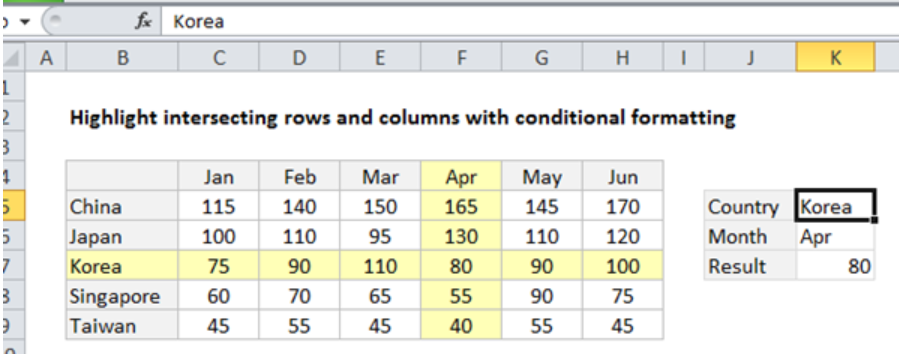Get instant live expert help with Excel or Google Sheets“My Excelchat expert helped me in less than 20 minutes, saving me what would have been 5 hours of work!”

#### Post your problem and you'll get expert help in seconds

Your message must be at least 40 characters
Our professional experts are available now. Your privacy is guaranteed.

# How to Highlight a Row and Column Intersection with Exact Match in Excel

It is now easy to highlight intersecting row and column in excel. This can be done by using a simple formula that is based on the OR function together with conditional formatting. This article will take you through the procedure of how to highlight intersecting rows and columns in excel.Figure 1. Final result

## General syntax of the formula

`=OR (\$A1=row_val,A\$1=col_val)`

## Explanation of the formula

The OR function plays a major role when it comes to helping us highlight the intersection point of a row and column. In the above example, we have the formula implemented as shown below;

`=OR(\$B4=\$K5,B\$4=\$K\$6)`

## Understanding how the formula works

For us to work with this formula to highlight intersecting rows and columns, we first need to apply conditional formatting relative to the active cell. In the case above, the active cell in our selection shall be B3. In this example, we shall use the formula below to highlight matching rows;

`\$B4=\$K\$5`

• Notice that in our example, reference B4 is mixed and the column is locked. This is to ensure that a comparison is only made between column B and country in cell K5.
• Also, note that the reference to cell K5 is absolute. This is so that changes are prevented when we apply conditional formatting.
• When applied, our rule should return TRUE for those cells that have “Korea”.
• The formula below shall help us highlight the matching column;

`B\$4=\$K\$6`

• In this case, cell B4 is locked. Unlike in the previous case, here the row is locked while the column is allowed to be relative. This allows us to make a comparison between values in row 4 to month values in cell K6.
• The cell K6 does not change during conditional formatting as it is absolute. In the above example, the formula will return TRUE for all the cells in the column where row is Apr.
• To trigger the CF above, we are supposed to wrap the formulas above in an OR function.
• The rule shall be triggered when either or both of the logicals bring back TRUE.

## Instant Connection to an Expert through our Excelchat Service

Most of the time, the problem you will need to solve will be more complex than a simple application of a formula or function. If you want to save hours of research and frustration, try our live Excelchat service! Our Excel Experts are available 24/7 to answer any Excel question you may have. We guarantee a connection within 30 seconds and a customized solution within 20 minutes.

Are you still looking for help with Conditional Formatting? View our comprehensive round-up of Conditional Formatting tutorials here.